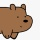Home
IT Knowledge
Inspiration
Languages
EN

# TypeScript - interface naming convention

31 points
Created by:Palus-Bear
646

This article explains the rules for interface naming convention used in TypeScript.

The interface naming convention defines the following rules:

1. Use PascalCase for interface names.
2. Use camelCase for interface members.
3. Do not use "I" as a prefix for interface names.
4. Use whole words in names when possible.

Example predefined interfaces: `Window`, `Document`, etc.

## Interface naming convention example

``````interface Square {

computePerimeter(aSize: number, bSize: number): number;
computeArea(aSize: number, bSize: number): number;

toString(): string;
}``````

Interface implementation:

``````class LocalSquare implements Square {

get name(): string {
return 'Square';
}

public computePerimeter(aSize: number, bSize: number): number {
return 2 * aSize + 2 * bSize;
}

public computeArea(aSize: number, bSize: number): number {
return aSize * bSize;
}

public toString(): string {
return `[ \${this.name} object ]`;
}
}``````

Run it online here.

## References

1. TypeScript Handbook Interface - Microsoft Github Docs